# NCERT Solutions for Class 8 Maths Exercise 3.2## myCBSEguide App

CBSE, NCERT, JEE Main, NEET-UG, NDA, Exam Papers, Question Bank, NCERT Solutions, Exemplars, Revision Notes, Free Videos, MCQ Tests & more.

NCERT Solutions for Class 8 Maths Exercise 3.2 Class 8 Maths book solutions are available in PDF format for free download. These ncert book chapter wise questions and answers are very helpful for CBSE exam. CBSE recommends NCERT books and most of the questions in CBSE exam are asked from NCERT text books. Class 8 Maths chapter wise NCERT solution for Maths Book all the chapters can be downloaded from our website and myCBSEguide mobile app for free.

NCERT solutions for Class 8 Maths Understanding Quadrilaterals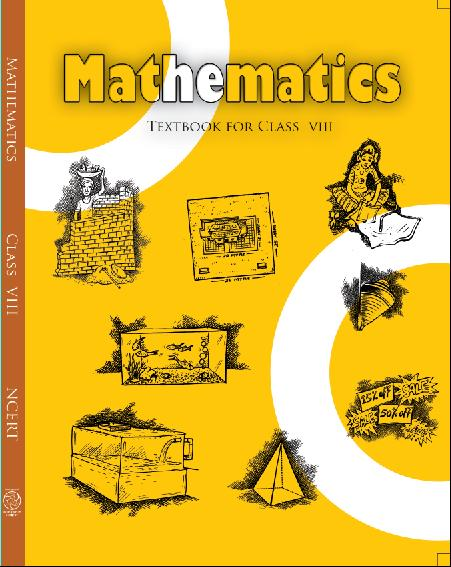## NCERT Solutions for Class 8 Maths Understanding Quadrilaterals

###### 1. Find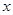in the following figures: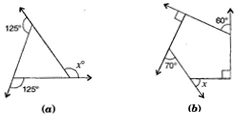Ans. (a) Here,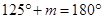[Linear pair]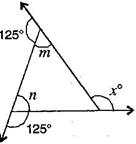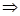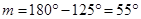And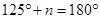[Linear pair]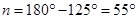{tex}\because {/tex} Exterior angle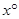= Sum of opposite interior angles

{tex}\therefore {/tex}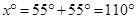(b) Sum of angles of a pentagon

=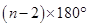=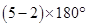=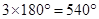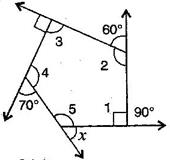By linear pairs of angles,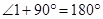……….(i)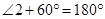……….(ii)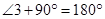……….(iii)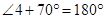……….(iv)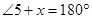……….(v)

Adding eq. (i), (ii), (iii), (iv) and (v),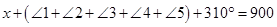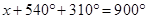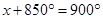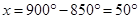NCERT Solutions for Class 8 Maths Exercise 3.2

###### 2. Find the measure of each exterior angle of a regular polygon of:

(a) 9 sides

(b) 15 sides

Ans. (i) Sum of angles of a regular polygon ==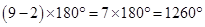Each interior angle =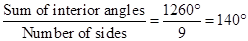Each exterior angle =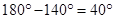(ii) Sum of exterior angles of a regular polygon =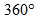Each interior angle =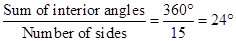###### 3. How many sides does a regular polygon have, if the measure of an exterior angle is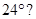Ans. Let number of sides be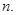Sum of exterior angles of a regular polygon =Number of sides =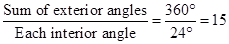Hence, the regular polygon has 15 sides.

NCERT Solutions for Class 8 Maths Exercise 3.2

###### 4. How many sides does a regular polygon have if each of its interior angles is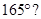Ans. Let number of sides beExterior angle =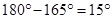Sum of exterior angles of a regular polygon =Number of sides =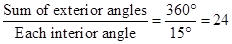Hence, the regular polygon has 24 sides.

###### 5. (a) Is it possible to have a regular polygon with of each exterior angle as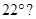(b) Can it be an interior angle of a regular polygon? Why?

Ans. (a) No. (Since 22 is not a divisor of)

(b) No, (Because each exterior angle is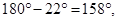which is not a divisor of)

NCERT Solutions for Class 8 Maths Exercise 3.2

###### 6. (a) What is the minimum interior angle possible for a regular polygon? Why?

(b) What is the maximum exterior angle possible for a regular polygon?

Ans. (a) The equilateral triangle being a regular polygon of 3 sides has the least measure of an

interior angle of {tex}{{60}^{\circ }}.{/tex}

{tex}\because {/tex} Sum of all the angles of a triangle

= {tex}180{}^\circ {/tex}

{tex}\therefore {/tex}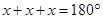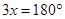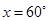(b) By (a), we can observe that the greatest exterior angle is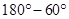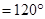.

## NCERT Solutions for Class 8 Maths Exercise 3.2

NCERT Solutions Class 8 Mathematics PDF (Download) Free from myCBSEguide app and myCBSEguide website. Ncert solution class 8 Mathematics includes text book solutions from Class 8 Maths Book . NCERT Solutions for CBSE Class 8 Maths have total 16 chapters. 8 Maths NCERT Solutions in PDF for free Download on our website. Ncert class 8 solutions PDF and Maths ncert class 8 PDF solutions with latest modifications and as per the latest CBSE syllabus are only available in myCBSEguide.

## CBSE app for Class 8

To download NCERT Solutions for class 8 Social Science, Computer Science, Home Science,Hindi ,English, Maths Science do check myCBSEguide app or website. myCBSEguide provides sample papers with solution, test papers for chapter-wise practice, NCERT solutions, NCERT Exemplar solutions, quick revision notes for ready reference, CBSE guess papers and CBSE important question papers. Sample Paper all are made available through the best app for CBSE students and myCBSEguide website.### 12 thoughts on “NCERT Solutions for Class 8 Maths Exercise 3.2”

1. Thanks Bro

2. really good app teacher

3. Thanks my cbse guide team.

4. It is so nice app

5. it is very good

6. it is very excllent

7. Thanks a lot
This was a question as home work individually

8. It’s very helpful

9. Good website of math I think

10. It is very helpful site for us because I complete my work very simply way??

11. thanks cbse guide team

12. Excellence ??